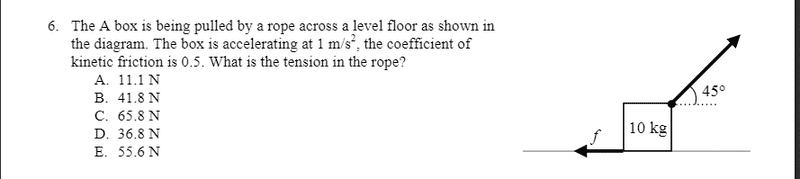# Find the tension in the rope pulling a box at an angle

• qwertyqwert321
In summary, the conversation is about a problem involving finding the tension force and normal force on a box on an inclined plane. The original attempt at a solution included incorrect equations for the vertical components of net force and frictional force, which were corrected by the expert. The student also found the tension force to be negative, which was incorrect. The expert requested for the student to show their working in order to determine where they went wrong.

## Homework Statement## The Attempt at a Solution

Here is what i have done so far:

Fx = cos 45 T - Ff =ma
Fy=sin 45 T -mg+ Fn =ma
I found ff= uk * Fn = 0.5 *sin 45 +mg
fn = sin 45 +mg

Isolated for T in the x and y:
Tx = ma + Ff / cos 45 = 13.404
Ty = ma +mg - Fn / sin 45 = 83.938

found the resultant for T: sqrt( (13.404)^2 + (83.938)^2 ) = 85 N?

What am i doing wrong? I've been stuck on this for 3 +hours now with no help :(

the answer is meant to be E.

Last edited:
qwertyqwert321 said:
Fy=sin 45 T -mg=ma
Presumably ##F_y## denotes the vertical component of net force on the box. That '=ma' should not be there. The acceleration is strictly horizontal, so ##a## shoud not appear in this equation about vertical components. Also, note that writing ##F_y## this way, in which the positive direction is up, it will be negative, because there cannot be a net upward force on the box, otherwise it would move upwards off the floor rather than horizontally along it.
I found ff= uk * Fn = 0.5 *sin 45 * mg
I don't know what 'uk' is. Assuming that 'ff' denotes frictional force, that force will be ##0.5F_y##, not what is written here.

andrewkirk said:
Presumably ##F_y## denotes the vertical component of net force on the box. That '=ma' should not be there. The acceleration is strictly horizontal, so ##a## shoud not appear in this equation about vertical components. Also, note that writing ##F_y## this way, in which the positive direction is up, it will be negative, because there cannot be a net upward force on the box, otherwise it would move upwards off the floor rather than horizontally along it.

I don't know what 'uk' is. Assuming that 'ff' denotes frictional force, that force will be ##0.5F_y##, not what is written here.
In the question, they stated the kinetic friction as 0.5 , which is what uk is.
and frictional force, ff, is uk * normal force (fn), therefore Ff = uk * Fn ? right?
I made the second equation as you said:

Fy=sin 45 T -mg+ Fn =0

solved for T and got -0.9998

i must be doing something wrong, I am just not sure what

qwertyqwert321 said:
solved for T and got -0.9998
We cannot tell where you went wrong if you do not post your working.
The two equations had up to that point look correct.

okay i now understand that I got the normal force equation wrong it is:
Fn = mg -T sin 45

why is the tension force negative? isn't Ty in the upward positive direction?

qwertyqwert321 said:
okay i now understand that I got the normal force equation wrong it is:
Fn = mg -T sin 45

why is the tension force negative? isn't Ty in the upward positive direction?
Yes, I understood that you had got that equation correct, but that still does not show me how you got from there to a wrong answer. Please show all your steps.